Definitions of Square Dance Calls and Concepts
Generous / Stingy CONCEPT [C3B]

Index -->  Plus  |  A1  |  A2  |  C1  |  C2  |  C3A  |  C3B  |  C4  |  NOL  |
Definitions (Text Only) -->  Plus  |  A1  |  A2  |  C1  |  C2  |  C3A  |  C3B  |  C4  |  NOL  |
 Find call:

 \$B8@8l(BStingy:
{anything} \$B%3!<%k\$r9T\$\$\$^\$9\$,(B, \$B%3!<%k\$N:G=i\$N(B Arm Turn \$B\$N2s\$kEY9g\$\$\$r(B 1/4 \$B8:\$i\$7\$^\$9(B.

Generous:
{anything} \$B%3!<%k\$r9T\$\$\$^\$9\$,(B, \$B%3!<%k\$N:G=i\$N(B Arm Turn \$B\$N2s\$kEY9g\$\$\$r(B 1/4 \$BA}\$d\$7\$^\$9(B.

\$B\$H(B Generous \$B\$NL\E*\$K\$h\$j(B, Arm Turn \$B\$O(B {anything} \$B\$N%3!<%k\$GDj5A\$5\$l\$F\$\$\$k(B, \$B2<5-\$NF0\$-\$K@)8B\$5\$l\$^\$9(B:

• Arm Turn.
• Mini-Wave \$B\$+\$i\$N(B Hinge.
• Mini-Wave \$B\$+\$i\$N(B Cast Off.
• \$B%*%]%8%C%H\$N?M\$H(B Mini-Wave \$B\$K\$J\$C\$F\$\$\$k\$H\$3\$m\$+\$i\$N(B Star turn.

\$BCm
• {anything} \$B\$N%3!<%k\$O(B Arm Turn \$B\$G;O\$^\$kI,MW\$O\$"\$j\$^\$;\$s(B; \$BNc\$(\$P(B, Generous Square Chain Thru (\$B8~\$+\$\$9g\$C\$?(B Line \$B\$+\$i\$N(B) \$B\$G\$O(B, Right Pull By \$B\$H(B 1/4 In; Left Arm Turn 3/4 (1/2 \$B\$NBe\$o\$j\$K(B); Centers Right Arm Turn 1/2; Left Arm Turn 1/2; Step Thru \$B\$r\$7\$^\$9(B. Trade By formation \$B\$G=*\$o\$j\$^\$9(B.
• Stingy \$B\$H(B Generous \$B\$O(B, \$B%3!<%k\$N:G=i\$N(B Arm Turn \$B\$K;H\$&\$3\$H\$G\$"\$j(B, \$B<+J,\$K\$H\$C\$F\$N;O\$a\$N(B Arm Turn \$B\$G\$O\$"\$j\$^\$;\$s(B. \$B\$"\$k?M\$K\$H\$C\$F\$O(B Arm Turn \$B\$rA4\$/\$7\$J\$\$\$G\$7\$g\$&\$7(B; \$B\$^\$?(B, \$BJL\$N?M\$,0[\$J\$k(B Arm Turn \$B\$r9T\$C\$?8e\$K(B Arm Turn \$B\$r\$9\$k\$3\$H\$b\$"\$j\$^\$9(B. \$B;O\$a\$N(B Arm Turn \$B\$r=*\$(\$?\$H\$-(B, \$B\$=\$N8e\$K\$"\$k(B Arm Turn \$B\$K\$D\$\$\$F\$O(B, Stingy \$B\$d(B Generous \$B\$O1F6A\$r5Z\$\\$7\$^\$;\$s(B.
• Stingy \$B\$H(B Generous \$B\$O(B, \$B%+%C%W%k\$+\$i\$N(B Hinge, Trade, \$B\$^\$?\$O(B Cast Off \$B\$K\$O;H\$\$\$^\$;\$s(B. \$BNc\$(\$P(B,

C3A \$B\$N%3!<%k(B Hinge The Lock \$B\$O(B, Hinge \$B\$r\$7\$F(B Lockit \$B\$G\$9(B. Generous Hinge The Lock \$B\$O(B (\$B>/\$J\$/\$H\$b(B) 2 \$B\$D\$N(B formation: Wave \$B\$^\$?\$O(B Tandem Couples (\$BLu

1. Wave \$B\$+\$i(B, Hinge \$B\$O(B Arm Turn 1/4 \$B\$G\$9(B. \$B\$7\$?\$,\$C\$F(B Wave \$B\$+\$i(B, Generous Hinge The Lock \$B\$O(B Arm Turn 1/2 \$B\$r\$7\$F(B Lockit \$B\$H\$J\$j\$^\$9(B.
2. Tandem Couples \$B\$+\$i\$O(B, Hinge \$B\$O(B Partner Hinge (\$B%+%C%W%k\$+\$i(B) \$B\$H\$J\$j(B, Generous \$B\$O;H\$\$\$^\$;\$s(B. \$B\$7\$+\$7\$J\$,\$i(B, Lockit \$B\$N(B Center \$B\$N%Q!<%H\$O(B Arm Turn 1/4 \$B\$G\$9(B. \$B\$7\$?\$,\$C\$F(B, Tandem Couples \$B\$+\$i\$N(B, Generous Lock The Hinge \$B\$O(B Partner Hinge \$B\$r\$7\$F(B, Centers \$B\$,(B Arm Turn 1/2 \$B\$r(B Ends \$B\$O(B move up 1/4 \$B\$r\$7(B, Facing Diamond \$B\$G=*\$o\$j\$^\$9(B.
• \$B\$b\$7(B, \$B%3!<%k\$N;O\$a\$N(B Arm Turn \$B\$,[#Kf\$N\$H\$-\$O(B, \$B%3!<%i!<\$OM>J,\$J8@MU\$r;H\$C\$F(B, \$B[#Kf\$5\$r\$J\$/\$9\$Y\$-\$G\$9(B: \$B\$9\$J\$o\$A(B, Points work Stingy: all Strip the Diamond \$B\$N\$h\$&\$G\$9(B. \$B\$^\$?(B, \$B%3!<%i!<\$O(B, Stingy \$B\$^\$?\$O(B Generous \$B\$r%3!<%k\$N\$I\$N%Q!<%H\$K;H\$&\$+\$r<(\$9\$?\$a\$K(B, Secondly \$B\$d(B Thirdly \$B\$J\$I\$N%3%s%;%W%H\$r;H\$&\$3\$H\$,\$G\$-\$^\$9(B.
• Stingy \$B\$H(B Generous \$B\$r;H\$&\$H(B, \$B;W\$\$\$b\$h\$i\$J\$\$(B formation \$B\$+\$i%3!<%k\$r9T\$&\$3\$H\$,\$G\$-\$^\$9(B. \$BNc\$(\$P(B, Twin Diamonds \$B\$+\$i(B: Generous Sets In Motion \$B\$,\$G\$-\$^\$9(B.
• Generous Dixie Style To A Wave: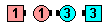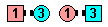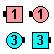\$B\$NA0(B Centers Right Pull By\$B\$N8e(B Left Touch 1/2\$B\$N8e(B (\$B=*\$o\$j(B)
Stingy Spin The Top: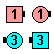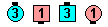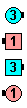\$B\$NA0(B Arm Turn 1/4\$B\$N8e(B Fan The Top\$B\$N8e(B (\$B=*\$o\$j(B)
Finally Stingy Spin The Top: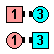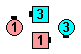\$B\$NA0(B Arm Turn 1/2\$B\$N8e(B Stingy Fan The Top\$B\$N8e(B (\$B=*\$o\$j(B)

\$BG'\$a\$i\$l\$J\$\$;H\$\$J}(B

• Generous Counter Rotate (Tidal Wave \$B\$+\$i(B): Very Center \$B\$N(B 2 \$B?M\$O(B, \$B\$*8_\$\$\$K(B 1/4 \$B2s\$j\$^\$9\$,(B, \$B%3!<%k\$O(B Arm Turn \$B\$d(B Hinge \$B\$N8@MU\$GDj5A\$5\$l\$F\$\$\$^\$;\$s(B.
• Generous Swap The Wave (\$B:8\$N(B Wave \$B\$+\$i(B): \$B\$"\$k?M\$O(B, center part \$B\$O(B Turn Thru (\$B\$9\$J\$o\$A(B Arm Turn) \$B\$G\$"\$k\$H
• Generous Pass The Ocean (\$B8~\$+\$\$9g\$C\$?%+%C%W%k\$+\$i(B:) Belle \$B\$N%Q!<%H\$O(B Left Arm Turm 1/4 \$B\$N\$h\$&\$K6/\$/46\$8(B, \$BIQHK\$K\$=\$N\$h\$&\$KMY\$i\$l\$F\$\$\$^\$9\$,(B, Pass The Ocean \$B\$NDj5A\$O(B Arm Turn \$B\$r4^\$s\$G\$\$\$^\$;\$s(B.
• Generous \$B\$H(B Stingy \$B\$NAH\$_9g\$o\$;\$K4X\$7\$F(B(\$BNc\$(\$P(B, Generous Stingy 1/4 Thru)... 2 \$B\$D\$N;vNc\$r9M\$(\$F\$_\$^\$7\$g\$&(B. Generous Stingy 1/4 Thru:

\$B;vNc(B 1.   Generous \$B\$H(B Stingy \$B\$O\$*8_\$\$\$KAj;&\$5\$l\$^\$9(B. Stingy \$B\$G;O\$a\$N(B Hinge \$B\$+\$i(B 1/4 \$B\$r8:\$i\$7(B, Generous \$B\$GA}\$d\$7\$F85\$XLa\$7\$^\$9(B. Stingy Arm Turn 1/4 \$B\$O(B, fraction \$B\$,(B 0/4 \$B\$G\$b(B Arm Turn \$B\$G\$9(B.

\$B;vNc(B 2.   Stingy 1/4 Thru \$B\$O(B Left Arm Turn 3/4 \$B\$G\$9(B (\$B;O\$a\$N(B Arm Turn \$B\$r\$d\$a\$F(B, Generous \$B\$r(B Left Arm Turn 1/2 \$B\$KEv\$F\$O\$a(B, Left Arm Turn 3/4 \$B\$H\$7\$^\$9(B).

\$B;vNc(B 2 \$B\$O?tG/A0\$K;n\$5\$l\$^\$7\$?\$,(B, \$B%@%s%5!<\$K\$OJ};O\$a\$N%Q!<%H\$K;H\$\$(B, \$B\$*8_\$\$\$KAj;&\$5\$l\$k!W\$G\$9(B. \$B\$3\$NOC\$N6571(B: Stingy \$B\$H(B Generous \$B\$rAH\$_9g\$o\$;\$J\$\$\$3\$H(B.

Yo-Yo Concept [C4]: \$BM?\$(\$i\$l\$?%3!<%k\$N;O\$a\$N(B Arm Turn 1/2 \$B\$r(B Arm Turn 3/4 \$B\$GCV\$-49\$(\$^\$9(B.

Fractal CONCEPT [C4] (Vic Ceder 1998): \$BM?\$(\$i\$l\$?%3!<%k\$N3F!9\$N(B Arm Turn 1/4 \$B\$r(B Arm Turn 3/4 \$B\$GCV\$-49\$((B, \$B5U\$K(B, \$B3F!9\$N(B Arm Turn 3/4 \$B\$r(B Arm Turn 1/4 \$B\$GCV\$-49\$(\$^\$9(B.Choreography for Generous / Stingy CONCEPTComments? Questions? Suggestions?

https://www.ceder.net/def/generous.php?level=C3B&language=japan
27-January-2020 05:59:35